# Physics Test 9 - Electromagnetic Waves, Optics, Modern Physics

## 25 Questions MCQ Test Mock Test Series for JEE Main & Advanced 2022 | Physics Test 9 - Electromagnetic Waves, Optics, Modern Physics

Description
Attempt Physics Test 9 - Electromagnetic Waves, Optics, Modern Physics | 25 questions in 60 minutes | Mock test for JEE preparation | Free important questions MCQ to study Mock Test Series for JEE Main & Advanced 2022 for JEE Exam | Download free PDF with solutions
QUESTION: 1

Solution:
QUESTION: 2

Solution:
QUESTION: 3

### In Young's double slit experiment, the fringe width is found to be 0.4 mm. If the whole apparatus is immersed in water of refractive index 4/3 without disturbing the geometrical arrangement, the new fringe width will be

Solution:
QUESTION: 4
For a prism of refractive index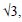the angle of the prism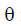is equal to the angle of minimum deviation.The value ofSolution:
QUESTION: 5
Two optical media of refractive indices n1 and n2 contain x and y waves of the same colour in the same thickness. Then their relative refractive index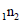is equal to
Solution:
QUESTION: 6
The focal length of a convex lens is 10 cm and its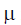is 1.5. If the radius of  curvature of one surface is 7.5 cm,the radius of curvature of the second surface will be
Solution:
QUESTION: 7
The refractive index of violet and red light are 1.54 and1.52 respectively. If the angle of prism is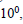the angular dispersion is
Solution:
QUESTION: 8
Interference fringes are obtained using two coherent sources whose intensities are in ratio 4 : 1. Then the ratio of the intensities of the bright and dark bands is
Solution:
QUESTION: 9
A ray of light passes through an equilateral prism such that the angle of incidence is equal to the angle of emergence and the latter equal to (3/4)th the angle of
the prism. The angle of deviation will be
Solution:
QUESTION: 10
A point object is placed at a distance of 10cm & its real image is formed at a distance of 20 cm from a concave mirror. If the object is moved by 0.1 cm towards the mirror, the image will shift by
Solution:
QUESTION: 11
Angle of a prism is A and its one surface is slivered.Light rays falling at an angle of incidence 2 A on first surface returns back, through, the same path after
suffering reflection at the second silvered surface.Refractive index of material is
Solution:
QUESTION: 12
Diamond shines due to its
Solution:
QUESTION: 13
When a beam of accelerated electrons hit a target a continuous X-ray spectrum is emitted from the target.Which one of these wavelengths is absent in the X-ray
spectrum if the X-rays tube is operated at 40,000V ?
Solution:
QUESTION: 14
The radio transmitter operates on a wavelength of 1500m at a power of 400 kilowatt. The energy of radiophoton in Joules is
Solution:
QUESTION: 15

What are the essential properties a medium must possess for the propagation of mechanical waves?

Solution:

The friction force amongst the particles of the medium should be negligibly small so that they continue oscillating for a sufficiently long time and the wave travels a sufficiently long distance through the medium

QUESTION: 16
The wavelength of the first line of Balmer series of hydrogen atom is l Å. The wavelength of this line of a double ionised lithium atom Z = 3) is
Solution:
QUESTION: 17
A freshly prepared radioactive source of half life 2 hrs emits radiations of intensity which is 64 times the permissible safe level. The minimum time after which it would be possible to work safely with this source is
Solution:
QUESTION: 18
A gamma ray photon creates an electron positron pair. If the rest mass energy of an electron is 0.5MeV and the total kinetic energy of the electron positron pair is 0.5 MeV, then the energy of the gamma ray photon must be
Solution:
QUESTION: 19
In the thermonuclear reaction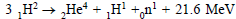the energy
released per nucleon of the reactants is
Solution:
QUESTION: 20

The relationship between α and β is given by

Solution:
*Answer can only contain numeric values
QUESTION: 21

A radiation energy 'E' falls normally on a perfectly reflecting surface. The momentum transferred to the surface is PE/C, then P is -

Solution:
*Answer can only contain numeric values
QUESTION: 22

Find the deviation of light ray (in degree)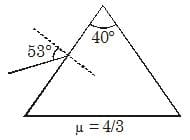Solution: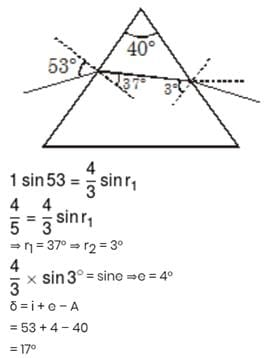*Answer can only contain numeric values
QUESTION: 23

In the arrangement shown a point source of light S is placed 60 cm to the left of a convex lens of focal length 30 cm and 2 cm above the common principal axis of the convex lens and a convex mirror of focal length 10 cm. Consider optic centre of the lens to be the origin, the x-coordinate of the final image of the source S for the viewer left to the lens is equal to (– K) cm. Calculate the value of K.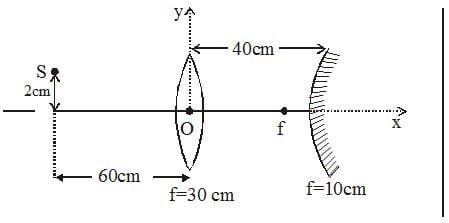Solution: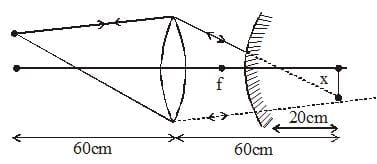*Answer can only contain numeric values
QUESTION: 24

An isotropic point source of light is kept at distance of 60 cm from a screen, the intensity of light obtained on center is I1. Now a lens of focal length 20 cm is introduced at exactly midway between source and screen and intensity obtained is I2. Find I2/8I1.

Solution: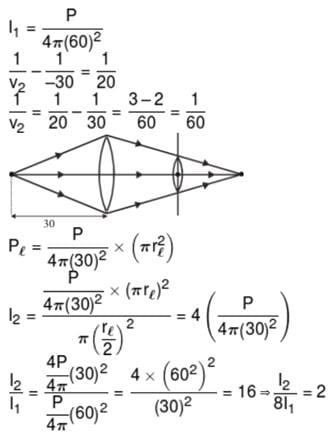*Answer can only contain numeric values
QUESTION: 25

A wave of frequency 100 Hz travels along a string towards its fixed end. When this wave travels back, after reflection, a node is formed at a distance of 10 cm from the fixed end. The speed of the wave (incident and reflected) is :

Solution: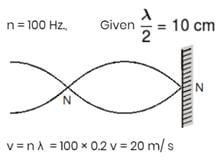Use Code STAYHOME200 and get INR 200 additional OFF Use Coupon Code